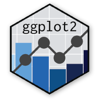Show Sidebar Hide Sidebar# Intro to Animations in ggplot2

How to create animations in ggplot2 with Plotly.

### New to Plotly?

Plotly's R library is free and open source!
You can set up Plotly to work in online or offline mode.
We also have a quick-reference cheatsheet (new!) to help you get started!

### Version Check

Version 4 of Plotly's R package is now available!
Check out this post for more information on breaking changes and new features available in this version.

``````library(plotly)
packageVersion('plotly')
``````
``````##  '4.9.1'
``````

### Frames

Now, along with `data` and `layout`, `frames` is added to the keys that `figure` allows. Your `frames` key points to a list of figures, each of which will be cycled through upon instantiation of the plot.

### Basic Example

``````library(plotly)

df <- data.frame(
x = c(1,2,3,4),
y = c(1,2,3,4),
f = c(1,2,3,4)
)

p <- ggplot(df, aes(x, y)) +
geom_point(aes(frame = f))

p <- ggplotly(p)

p
``````

### Mulitple Trace Animations

``````library(plotly)
library(gapminder)

p <- ggplot(gapminder, aes(gdpPercap, lifeExp, color = continent)) +
geom_point(aes(size = pop, frame = year, ids = country)) +
scale_x_log10()

p <- ggplotly(p)

p
``````

``````library(plotly)

p <- p %>%
animation_opts(
1000, easing = "elastic", redraw = FALSE
)

p
``````

``````library(plotly)